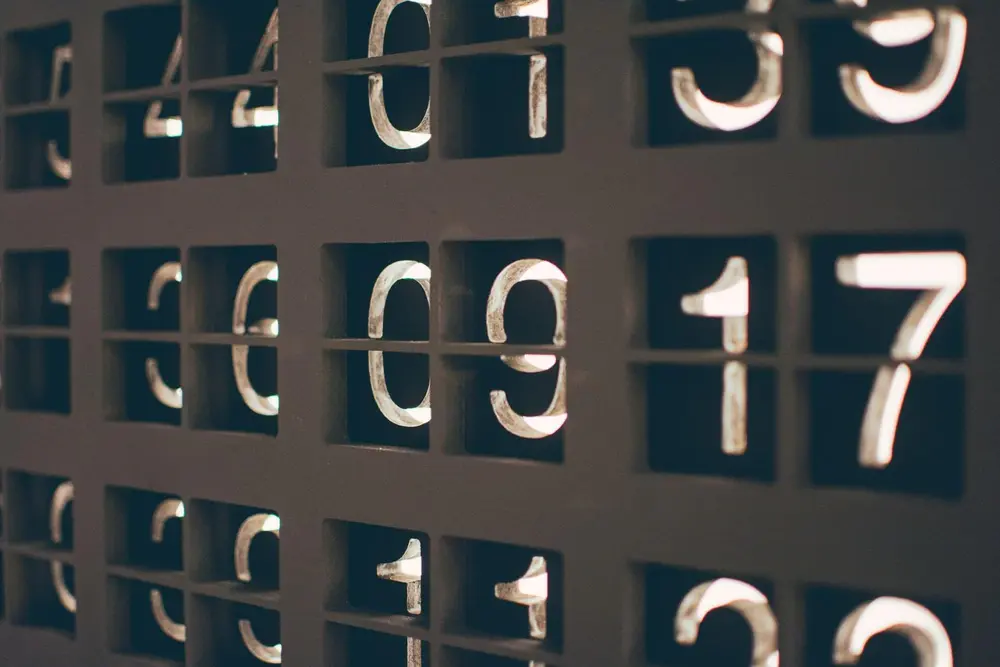# Numbers

• Without numbers, mathematics cannot exist. There are a variety of numbers which you are required to know about.
• Real Numbers - These are rational and irrational numbers.
• Rational Numbers - These will always have terminating or recurring decimals. This means they can always be turned into a fraction.
• Irrational Numbers - These can not be turned into a fraction. They are non-terminating.
• Integers - These are whole positive or negative numbers (-3, -2, -1, 0, 1, 2, 3).
• Prime Numbers - These are numbers with only TWO factors, itself and 1.
• Square Numbers - These numbers are formed by a number multiplied by itself (1, 4, 9, 16...).
Number

Number

Number

Number

Number

Number